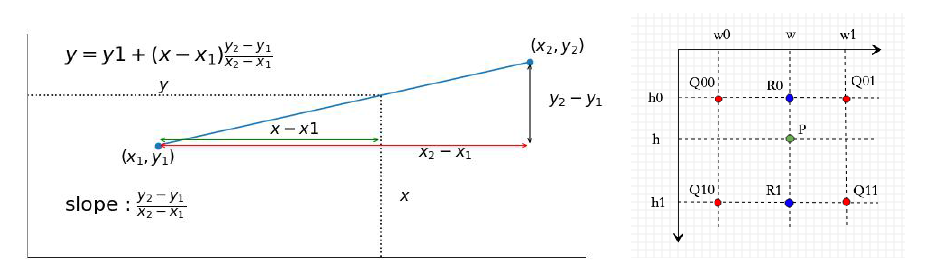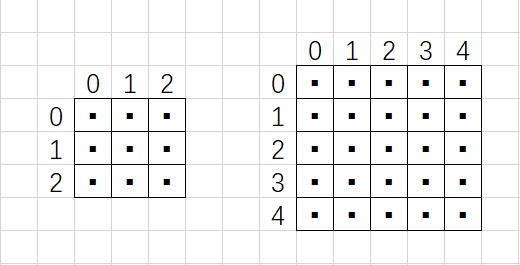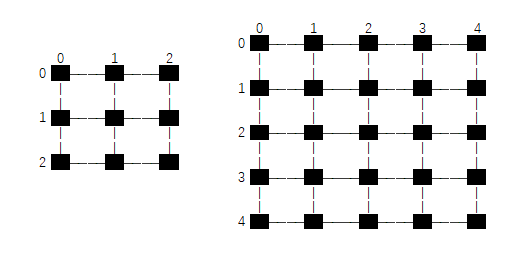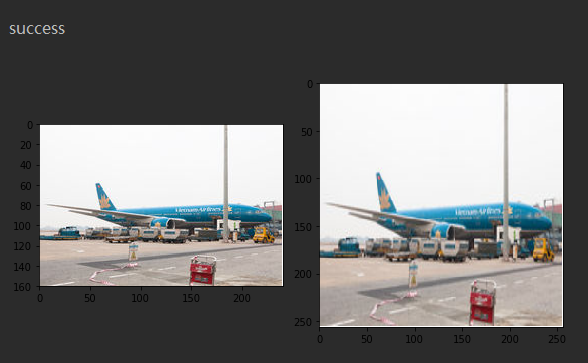• c# 反距离加权插值+临近差值+双线性差值，用窗体程序实现，可以看到效果图片
• 很好的几种图像缩放算法。包括采用临近点 线性差值 三次样条差值 三次卷积差值四种方法非常强悍。
• 自己写的基于科学出版社的朱虹老师数字图像处理一些简单的数字图像处理代码，主要包含有：K均值去噪，直方图均衡化，临近插值旋转，双线性差值放大，双线性差值旋转，中值滤波，线性展宽等代码。希望对大家有用！
• 然后记录每个桶中的最大值和最小值，最大差值在两个临近桶之间。 推荐看左神视频初级班第三讲100分钟左右 考查内容 桶排序 #include<bits/stdc++.h> using namespace std; const int INF = 0x3fffffff...
题目链接
时间复杂度$O(n)$
利用n个数放在n+1个桶中，必然有一个空桶，这也就否定了最大差值在桶内部。
然后记录每个桶中的最大值和最小值，最大差值在两个临近桶之间。
推荐看左神视频初级班第三讲100分钟左右
考查内容
桶排序
#include<bits/stdc++.h>
using namespace std;

const int INF = 0x3fffffff;
typedef long long LL;
LL m,n;
vector<LL> arr;

LL bucket(LL x,LL Max, LL Min){
return (x - Min)*n/(Max - Min);
}
int main(){
while(cin>>m){
LL Max = -INF, Min = INF;
LL x;
char c;
while(cin>>x){
arr.push_back(x);
if(x > Max) Max = x;
if(x < Min) Min = x;
c = getchar();
if(c =='\n') break;
}
n = arr.size();
if(Max == Min || n < 2){
cout<<0<<endl;
continue;
}
//n+1个桶中放n个数
vector<bool> hasNum(n+1); //标记桶的状态
vector<LL> maxs(n+1),mins(n+1); //记录桶中的最大值和最小值
LL bid;
for(LL i = 0; i < n; i++){
bid = bucket(arr[i], Max, Min);
maxs[bid] = hasNum[bid]? max(maxs[bid], arr[i]) : arr[i]; //保存最大值
mins[bid] = hasNum[bid]? min(mins[bid], arr[i]) : arr[i];//保存最小值
hasNum[bid] = true;
}
LL res = 0;
LL lastMax = maxs;

for(LL i = 1; i <= n; i++){ //n+1个桶
if(hasNum[i]){
res = max(res, mins[i] - lastMax); //全部桶里面找
lastMax = maxs[i];
}
}
cout<<res<<endl;
}
return 0;
}



展开全文• 所谓插值，是用于图像放大的一种图像处理方式，临近插值是最简单最常用的放大方式之一，很多图像放大算法都是临近差值。若想将 的图片放大为 ， 则其计算公式为：  其中为 目标图片的轴像素，...
写作动机

最近写打马赛克的算法，查了很多博客，用的都是左上角元素填充。 通过各种搜索和计算，最终总结发现了一个规律： 马赛克边长为色块区域边长的整数倍时，临近采样后的结果等同于用左上角元素填充。

知识背景

所谓插值，是用于图像放大的一种图像处理方式，临近插值是最简单最常用的放大方式之一，很多图像放大算法都是临近差值。若想将  的图片放大为 ， 则其计算公式为：

其中为 目标图片的轴像素， 为原图片的轴像素。

在做打马赛克的算法时，用左上角像素填充色块算是临近采样的一种。 无论是采样还是插值，其根本思想是临近的概念。总的来说，意思就是：目标图片的目标像素要取原图像的哪个像素呢？ 目标像素后 取整。

结论：

设  ，若， 即目标图片边长为色块区域边长的整数倍时，临近采样后的结果等同于用左上角元素填充。

证明如下：

原图：

a
b
c
d
目标图片（假设）：

a
_
_
b
_
_
_
_
_
_
_
_
_
_
_
_
_
_
c
_
_
d
_
_
_
_
_
_
_
_
_
_
_
_
_
_

在插值的时候，

y轴同理，因此最终的临近插值结果为：

a
a
a
b
b
b
a
a
a
b
b
b
a
a
a
b
b
b
c
c
c
d
d
d
c
c
c
d
d
d
c
c
c
d
d
d

展开全文• 通过处理， 模糊图像变的清晰自己可以修改下引用的位置，自己编的，运行过希望对大家有用
• 在理解双线性差值（Bilinear Interpolation）的含义基础上，参考pytorch差值的官方实现注释，自己实现了一遍。共三段代码： 一、for循环实现双线性差值（naive实现） 是对一张图像的，维度HWC，采用for循环遍历H...
一、双线性差值

1.1 公式

在理解双线性差值（Bilinear Interpolation）的含义基础上，参考pytorch差值的官方实现注释，自己实现了一遍。

差值就是利用已知点来估计未知点的值。一维上，可以用两点求出斜率，再根据位置关系来求插入点的值。同理，在二维平面上也可以用类似的办法来估计插入点的值。如图，已知四点、、、四点的值与坐标值、、、，求位于的点的值。思路是

先用w方向一维的线性差值，根据、求出点，根据、求出点；
再用h方向一维线性差值，根据和求出点；
那么就有如下公式

具体到图像的双线性差值问题，我们可以理解成将图片进行了放大，但不使图像变成大块的斑点状，而是增大了图像的分辨率，多出来的像素就是双线性差值的结果。图像上周边4点一定是临近的，也就是说

上面的公式简化为

这样我们就面临将目标图像的坐标映射到原图像上求出的问题。

1.2 坐标变换

对于第一个问题,目标图像的坐标映射到原图像上求出，有两种思路。

第一种是把像素点看成是1×1大小的方块，像素点位于方块的中心，坐标转换时，HW方向的坐标都要加0.5才能对应起来。pytorch里面叫做torch.nn.functional.interpolate(align_corners=False)。

举例，如图原图像是一个3×3的图像，放大到5×5，每个像素点都是位于方形内的黑色小点。设是原图像的大小，本例是3×3，是目标图像的大小，本例是5×5。换算公式为第二种是上下左右相邻的像素点之间连线，像素点都位于交点上，坐标转换时，HW方向的总长度都要减少1才能对应起来g。pytorch里面叫做torch.nn.functional.interpolate(align_corners=True)。

举例，一个3×3的图像放大到5×5，每个像素点都是位于交点的黑色小点。设是原图像的大小，本例是3×3，是目标图像的大小，本例是5×5。换算时，我们取边的长度，也就是HW方向各减1，也就是从2×2变成4×4。这样就有个结论就是变换以后目标图像四个顶点的像素值一定和原图像四个顶点像素值一样。换算公式为二、for循环实现双线性差值（naive实现）

是对一张图像的，维度HWC，采用for循环遍历H、W计算差值点的像素值。这个实现too young，too simple，简直naive，效率低但易于理解；这里只实现了第一种坐标变换。

import matplotlib.pyplot as plt
import matplotlib.image as mpimg
import numpy as np
import os

def bilinear_interpolation_naive(src, dst_size):
"""
双线性差值的naive实现
:param src: 源图像
:param dst_size: 目标图像大小H*W
:return: 双线性差值后的图像
"""
(src_h, src_w, src_c) = src.shape  # 原图像大小 H*W*C
(dst_h, dst_w), dst_c = dst_size, src_c  # 目标图像大小H*W*C

if src_h == dst_h and src_w == dst_w:  # 如果大小不变，直接返回copy
return src.copy()

scale_h = float(src_h) / dst_h  # 计算H方向缩放比
scale_w = float(src_w) / dst_w  # 计算W方向缩放比
dst = np.zeros((dst_h, dst_w, dst_c), dtype=src.dtype)  # 目标图像初始化
for h_d, row in enumerate(dst):  # 遍历目标图像H方向
for w_d, col in enumerate(row):  # 遍历目标图像所有W方向
h = scale_h * (h_d + 0.5) - 0.5  # 将目标图像H坐标映射到源图像上
w = scale_w * (w_d + 0.5) - 0.5  # 将目标图像W坐标映射到源图像上
h0 = int(np.floor(h))  # 最近4个点坐标h0
w0 = int(np.floor(w))  # 最近4个点坐标w0
h1 = min(h0 + 1, src_h - 1)  # h0 + 1就是h1，但是不能越界
w1 = min(w0 + 1, src_w - 1)  # w0 + 1就是w1，但是不能越界
r0 = (w1 - w) * src[h0, w0, ...] + (w - w0) * src[h0, w1, ...]  # 双线性差值R0
r1 = (w1 - w) * src[h1, w0, ...] + (w - w0) * src[h1, w1, ...]  # 双线性插值R1
p = (h1 - h) * r0 + (h - h0) * r1  # 双线性插值P
dst[h_d, w_d, ...] = p.astype(np.uint8) # 插值结果放进目标像素点
return dst

if __name__ == '__main__':
def unit_test():
image_file = os.path.join(os.getcwd(), 'test.jpg')
image_scale = bilinear_interpolation_naive(image, (256, 256))

fig, axes = plt.subplots(1, 2, figsize=(8, 10))
axes = axes.flatten()
axes.imshow(image)
axes.imshow(image_scale)
axes.axis([0, image.shape, image.shape, 0])
axes.axis([0, image_scale.shape, image_scale.shape, 0])
fig.tight_layout()
plt.show()
pass

unit_test()



三、用numpy矩阵实现

是对一张图像的，维度HWC；采用numpy矩阵实现，速度快；

import matplotlib.pyplot as plt
import matplotlib.image as mpimg
import numpy as np
import os
import torch

def bilinear_interpolation(src, dst_size, align_corners=False):
"""
双线性插值高效实现
:param src: 源图像H*W*C
:param dst_size: 目标图像大小H*W
:return: 双线性插值后的图像
"""
(src_h, src_w, src_c) = src.shape  # 原图像大小 H*W*C
(dst_h, dst_w), dst_c = dst_size, src_c  # 目标图像大小H*W*C

if src_h == dst_h and src_w == dst_w:  # 如果大小不变，直接返回copy
return src.copy()
# 矩阵方式实现
h_d = np.arange(dst_h)  # 目标图像H方向坐标
w_d = np.arange(dst_w)  # 目标图像W方向坐标
if align_corners:
h = float(src_h - 1) / (dst_h - 1) * h_d
w = float(src_w - 1) / (dst_w - 1) * w_d
else:
h = float(src_h) / dst_h * (h_d + 0.5) - 0.5  # 将目标图像H坐标映射到源图像上
w = float(src_w) / dst_w * (w_d + 0.5) - 0.5  # 将目标图像W坐标映射到源图像上

h = np.clip(h, 0, src_h - 1)  # 防止越界，最上一行映射后是负数，置为0
w = np.clip(w, 0, src_w - 1)  # 防止越界，最左一行映射后是负数，置为0

h = np.repeat(h.reshape(dst_h, 1), dst_w, axis=1)  # 同一行映射的h值都相等
w = np.repeat(w.reshape(dst_w, 1), dst_h, axis=1).T  # 同一列映射的w值都相等

h0 = np.floor(h).astype(np.int)  # 同一行的h0值都相等
w0 = np.floor(w).astype(np.int)  # 同一列的w0值都相等

h0 = np.clip(h0, 0, src_h - 2)  # 最下一行上不大于src_h - 2，相当于padding
w0 = np.clip(w0, 0, src_w - 2)  # 最右一列左不大于src_w - 2，相当于padding

h1 = np.clip(h0 + 1, 0, src_h - 1)  # 同一行的h1值都相等，防止越界
w1 = np.clip(w0 + 1, 0, src_w - 1)  # 同一列的w1值都相等，防止越界

q00 = src[h0, w0]  # 取每一个像素对应的q00
q01 = src[h0, w1]  # 取每一个像素对应的q01
q10 = src[h1, w0]  # 取每一个像素对应的q10
q11 = src[h1, w1]  # 取每一个像素对应的q11

h = np.repeat(h[..., np.newaxis], dst_c, axis=2)  # 图像有通道C，所有的计算都增加通道C
w = np.repeat(w[..., np.newaxis], dst_c, axis=2)
h0 = np.repeat(h0[..., np.newaxis], dst_c, axis=2)
w0 = np.repeat(w0[..., np.newaxis], dst_c, axis=2)
h1 = np.repeat(h1[..., np.newaxis], dst_c, axis=2)
w1 = np.repeat(w1[..., np.newaxis], dst_c, axis=2)

r0 = (w1 - w) * q00 + (w - w0) * q01  # 双线性插值的r0
r1 = (w1 - w) * q10 + (w - w0) * q11  # 双线性差值的r1
q = (h1 - h) * r0 + (h - h0) * r1  # 双线性差值的q
dst = q.astype(src.dtype)  # 图像的数据类型
return dst

if __name__ == "__main__":
def unit_test2():
image_file = os.path.join(os.getcwd(), 'test.jpg')
image_scale = bilinear_interpolation(image, (256, 256))

fig, axes = plt.subplots(1, 2, figsize=(8, 10))
axes = axes.flatten()
axes.imshow(image)
axes.imshow(image_scale)
axes.axis([0, image.shape, image.shape, 0])
axes.axis([0, image_scale.shape, image_scale.shape, 0])
fig.tight_layout()
plt.show()
pass

unit_test2()

def unit_test3():
src = np.array([[1, 2], [3, 4]])
print(src)
src = src.reshape((2, 2, 1))
dst_size = (4, 4)
dst = bilinear_interpolation(src, dst_size)
dst = dst.reshape(dst_size)
print(dst)

tsrc = torch.arange(1, 5, dtype=torch.float32).view(1, 1, 2, 2)
print(tsrc)
tdst = F.interpolate(
tsrc,
size=(4, 4),
mode='bilinear'
)
print(tdst)
# unit_test3()


四、用torch张量实现

是对tensor的，维度NCHW；和第二段一样，但是采用了张量，可以批量处理。

import torch
import torch.nn.functional as F
import os
import matplotlib.pyplot as plt
import matplotlib.image as mpimg

def bilinear_interpolate(src, dst_size, align_corners=False):
"""
双线性差值
:param src: 原图像张量 NCHW
:param dst_size: 目标图像spatial大小(H,W)
:param align_corners: 换算坐标的不同方式
:return: 目标图像张量NCHW
"""
src_n, src_c, src_h, src_w = src.shape
dst_n, dst_c, (dst_h, dst_w) = src_n, src_c, dst_size

if src_h == dst_h and src_w == dst_w:
return src.copy()
"""将dst的H和W坐标映射到src的H和W坐标"""
hd = torch.arange(0, dst_h)
wd = torch.arange(0, dst_w)
if align_corners:
h = float(src_h - 1) / (dst_h - 1) * hd
w = float(src_w - 1) / (dst_w - 1) * wd
else:
h = float(src_h) / dst_h * (hd + 0.5) - 0.5
w = float(src_w) / dst_w * (wd + 0.5) - 0.5

h = torch.clamp(h, 0, src_h - 1)  # 防止越界，0相当于上边界padding
w = torch.clamp(w, 0, src_w - 1)  # 防止越界，0相当于左边界padding

h = h.view(dst_h, 1)  # 1维dst_h个，变2维dst_h*1个
w = w.view(1, dst_w)  # 1维dst_w个，变2维1*dst_w个
h = h.repeat(1, dst_w)  # H方向重复1次，W方向重复dst_w次
w = w.repeat(dst_h, 1)  # H方向重复dsth次，W方向重复1次

"""求出四点坐标"""
h0 = torch.clamp(torch.floor(h), 0, src_h - 2)  # -2相当于下边界padding
w0 = torch.clamp(torch.floor(w), 0, src_w - 2)  # -2相当于右边界padding
h0 = h0.long()  # torch坐标必须是long
w0 = w0.long()  # torch坐标必须是long

h1 = h0 + 1
w1 = w0 + 1

"""求出四点值"""
q00 = src[..., h0, w0]
q01 = src[..., h0, w1]
q10 = src[..., h1, w0]
q11 = src[..., h1, w1]

"""公式计算"""
r0 = (w1 - w) * q00 + (w - w0) * q01  # 双线性插值的r0
r1 = (w1 - w) * q10 + (w - w0) * q11  # 双线性差值的r1
dst = (h1 - h) * r0 + (h - h0) * r1  # 双线性差值的q

return dst

if __name__ == '__main__':
def unit_test4():
# src = torch.randint(0, 100, (1, 3, 3, 3))
src = torch.arange(1, 1 + 27).view((1, 3, 3, 3))\
.type(torch.float32)
print(src)
dst = bilinear_interpolate(
src,
dst_size=(4, 4),
align_corners=True
)
print(dst)
pt_dst = F.interpolate(
src.float(),
size=(4, 4),
mode='bilinear',
align_corners=True
)
print(pt_dst)
if torch.equal(dst, pt_dst):
print('success')

image_file = os.path.join(os.getcwd(), 'test.jpg')

image_in = torch.from_numpy(image.transpose(2, 0, 1))
image_in = torch.unsqueeze(image_in, 0)
image_out = bilinear_interpolate(image_in, (256, 256))
image_out = torch.squeeze(image_out, 0).numpy().astype(int)
image_out = image_out.transpose(1, 2, 0)

fig, axes = plt.subplots(1, 2, figsize=(8, 10))
axes = axes.flatten()
axes.imshow(image)
axes.imshow(image_out)
axes.axis([0, image.shape, image.shape, 0])
axes.axis([0, image_out.shape, image_out.shape, 0])
fig.tight_layout()
plt.show()

unit_test4()展开全文AI
• 最邻近差值 方法介绍： 最邻近差值是做什么的？这个方法是用来放大图像的，这个方法需要我们指定倍率，运用差值公式，就可以计算出新的图像啦 上式为插值公式，a表示...但是，第一次学习临近插值时我会有这样的疑问
最邻近差值
方法介绍：
最邻近差值是做什么的？这个方法是用来放大图像的，这个方法需要我们指定倍率，运用差值公式，就可以计算出新的图像啦上式为插值公式，a表示放大倍数，I表示原始图像，I表示被放大之后的图片，（x,y）的组合表示了一个确定的像素值
但是具体怎么实现，还是需要将细节挪列出来
大家想一想，在将一张图像进行放大之前，我们应该先做的一件事情就是构造好放大之后的模板矩阵I（但是现在，这个矩阵里还是什么都没有），所以现在我们要做的事情就是构造这个模版矩阵
但是，第一次学习临近插值时我会有这样的疑问：就算模版矩阵构造好了，那怎么将模版矩阵里的值与原始图像的像素进行一一对应呢？
用「下标索引」，创建好的模版矩阵里面并不是什么都没有，而是记录了放大之后的行、列信息。
所以，为了方便的记录每一个点的行列信息，我们打算创建两个矩阵，一个用来记录行值信息，另一个用来记录列值信息，把他们分别保存起来，如下图（PS:只是举例说明分开储存信息让接下来的一一对应更加方便，不用纠结图片是怎么来的）：
y_index: 列值信息x_inedx:行值信息好了，到这一步为止，最难的部分我觉得已经解决了，接下来就是要组合行列信息（x_index, y_index），将两个矩阵相同位置的值组合起来，最后将组合好的下标对应到原始图像上去。
等等，还有最后一个问题
例如原始图像的大小是36x36，需要放大2倍，得到72x72，那么行列矩阵组合之后一定会有（72，72）（71，71）等等这些像素，如何把他们对应起来呢？这里需要考虑两个问题：
1）运用差值公式，如文章开头：
所以（72, 72）–>(72/2, 72/2)–>(36, 36)完成对应
2）得到小数怎么办？
（71/2，71/2）–>(35.5, 35.5)，这个时候就四舍五入吧 -->(36, 36)
实现代码如下：

import cv2 as cv
import numpy as np

def nn_outsize(img, ah=1, aw=1):
h, w, c = img.shape

new_h = int(h * ah)
new_w = int(h * aw)

# 得到新的两个下标矩阵
x_index = np.arange(new_h).repeat(new_w).reshape(new_w, -1)
y_index = np.tile(np.arange(new_w), (new_h, 1))

# 差值公式
x_index = np.round(x_index / ah).astype(np.int)
y_index = np.round(y_index / aw).astype(np.int)
# 映射
out = img[x_index, y_index]

return out

out = nn_outsize(img, ah=1.5, aw=1.5)
cv.imshow('nn_inter', out)
cv.waitKey(0)

说明：
1）a.repeat(num)将a数组中的每个元素重复num次
2）reshape(x, -1)表示根据x的维度来确定另一个方阵
3）np.arange()可以用来创建数组（起始， 末尾， 步长）
4）np.tile()对整个数组拼接，tile（数组，（行，列））以（行，列）为格式复制数组。
最后总结一下思路：
1）读取图像的原始长、宽、频道，并给出放大比例
2）计算被放大后的图像的原始矩阵（矩阵内值未确定）
a.计算下标为横坐标的矩阵
b.计算下标为纵坐标的矩阵
3）运用插值公式，将原始图像的坐标与计算出的差值坐标相对应
但是也会出现问题：
当我修改ah=3, aw=3想放大3倍时报错（原图为128x128）烦！
参考资料：图像100问之问题二十五：最邻近插值


展开全文• 本篇文章主要内容：k-临近分类算法概述从文本文件中解析和导入数据 使用Matplotlib创建扩散图归一化数据一、k-临近算法概述 简单地说，k-临近算法采用测量不同特征值之间的距离方法进行分类工作原理： 存在一个...机器学习
• x=0:2*pi;y=sin(x);xx=0:0.5:2*pi;%interp1对sin函数进行分段线性插值，调用interp1的时候...figureplot(x,y,'o',xx,y1,'r')title('分段线性插值')%临近插值y2=interp1(x,y,xx,'nearest');figureplot(x,y,'o',xx,y2,...
• 最邻近差值算法的原理：查找像素点最近的点进行像素拷贝伪代码insert_near (src, dst){ wscale = src.width/dst.width; //得出宽度比 hscale = src.height/dst.height; //得到高度比 for(dy = 0; dy sy = dy * ...
• 可以任意缩放yuv，rgb565，bmp格式图片，生成图片在当前目录。缩放算法支持最临近差值和双线性内差值。如果不选算法，则将图像转成bmp文件，用于查看ycbcr和rgb565的图像。
• 这样， 每次取mid时会更加接近targe值（比较targe和边界值的大小，进而让mid更加接近临近的边界下标） 代码： package Search; public class InsertValueSearch { //差值查找（只是mid定义变了，变成了mid = left +...算法 查找算法
• MySql计算两日期时间之间相差的天数,秒数,分钟数,周数,小时数 计算两日期时间之间相差的天数，秒数，分钟数，周数，小时数，这里主要分享的是通过MySql内置的函数 TimeStampDiff() 实现。 函数 TimeStampDiff() 是...mysql
• 也就是根据三维坐标计算距离周围格子的距离，按距离的倒数计算权重，将梯度幅值按权重分配到临近的格子里。 3. 附 zddhub 的一幅图    * 注意： 在(3)式进行计算(x'',y'')时，会减去0.5, 主要是...
• 此外,该有两个点需要说明,一个是为了防止各维度数据对结果的影响不均匀(例如A维度的差值是100,B维度的差值是0.01,A维度对结果的影响程度明显远高于B维度,但是在事实上A,B维度应该是相同的),我们可以对所有维度的距离...分类
• 原因：yolov4中存网络中使用临近差值上采样在tensorRT中不支持。 解决方案： 修改yolov4网络模型中的上采样，使用下面的代码代替网络中的torch.nn.Upsample import torch import torch.nn as nn import torch.nn....pytorch 深度学习
• 最简单的是最临近插值算法，这种算法就是根据原图像和目标图像的尺寸，计算缩放的比例，然后根据缩放比例计算目标像素所依据的原像素，过程中自然会产生小数，这时就采用四舍五入，取与这个点最相近的点。...图像处理 最邻近插值
• ’nearest’：最临近插值； ’spline’：三次样条插值； ’cubic’：双三次插值。 例2-33： >>[X,Y] = meshgrid(-3:.25:3); >>Z = peaks(X,Y); >>[XI,YI] = meshgrid(-3:.125:3); ...
• 比如 double a[]={1,5,4,0.2,100} 这个无序的数组，相邻的数的最大差值为100-5=95. 题目分析：这题有个简单的做法，首先就是对数组进行一个排序，然后扫面一遍数据就可以得到结果；但时间复杂度依赖于排序时间...算法
• ## BMP的图像处理

千次阅读 2016-01-13 15:33:10
最近碰到了一个问题将图片缩放: ...进行图片缩放的时候会进行一些处理（最临近差值法）： 具体的代码如下： #include #include #include #include #include LONG IWidth; //原图像的宽 LONG IHeight; /位图 结构
• ## K临近算法

千次阅读 2015-03-26 10:02:31
diffMat = tile(inputPoint,(dataSetSize,1))-dataSet #样本与训练集的差值矩阵 sqDiffMat = diffMat ** 2 #差值矩阵平方 sqDistances = sqDiffMat.sum(axis=1) #计算每一行上元素的和 distances = ...机器学习
• 使用Peter的机器学习实战学习了knn...最多的那个则为对应的标签使用书上0-9数据集(每个数字都有接近200个样本)进行训练和测试的时候发现，k为3时最佳，k变大时错误率会上升，越大错的越多因为数据的某两个属性的差值...
• 工作原理：存在一个样本数据集合，也称为训练样本集，并且样本集中每个...一般来说，我们只选择样本数据集中前k个最相似数据，这就是k-临近算法的出处，通常k是不大于20的整数。最后选择k个最相似数据中出现次数最多机器学习 python
• #对应的差值 sqDiffMat = diffMat** 2 #差的平方 sqDistance = sqDiffMat.sum(axis= 1 ) #差的平方的和 distance = sqDistance ** 0.5 #差的平方的和的平方根 sortedDistIndicies = distance.argsort() #...
• 最邻近插值算法和双线性插值算法——图像缩放 加载图像时经常会遇见要缩放图像的情况，这种时候如何决定缩放后图像对应像素点的像素值，这时候就需要用到插值算法 1.最邻近插值算法 首先假设原图是一个像素大小为WH...
• 图像缩放的最临近插值算法的原理解析 图像的缩放很好理解,就是图像的放大和缩小。传统的绘画工具中,有一种叫做“放大尺”的绘画工具，画家常用它来放大图画。当然，在计算机上，我们不再需要用放大尺去放大或缩小...
• 图像放缩之临近点插值 一：数学原理 当一幅二维数字图像从源图像N*M被放为(j*N) * (k*M)目标图像是，参照数学斜率计算公式 必然有: (X1 – Xmin)/(Xmax - Xmin) = (Y1 - Ymin)/(Ymax-Ymin)   ...图像处理 c java...